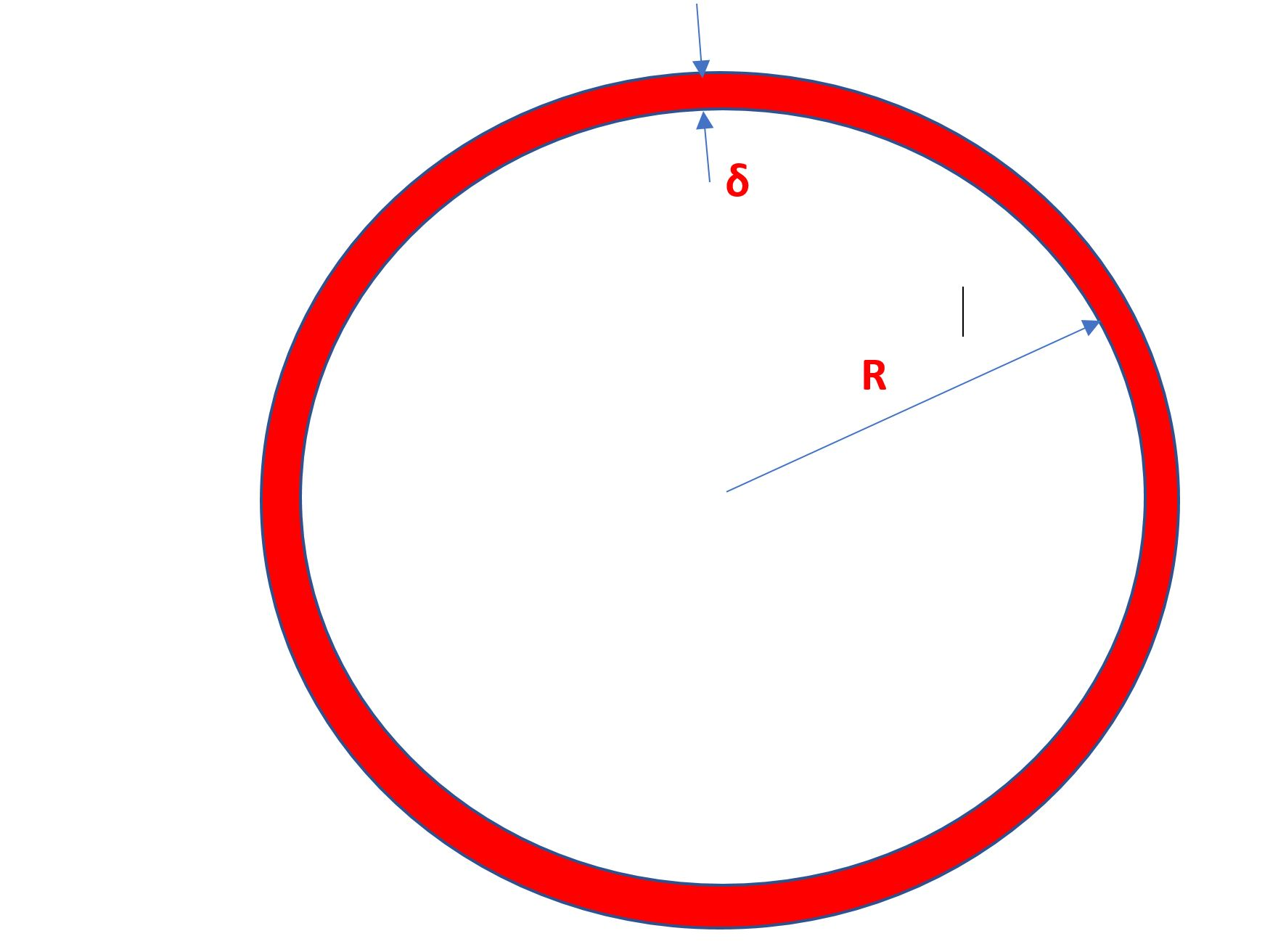# Mr. Olbers and his paradoxWhy is the night sky dark? Wilhelm Olbers asked this question, certainly not for the first time in history, in the 1800s.

That’s a silly question with an obvious answer. Isn’t that so?

Let’s see. There certainly is no sun visible, which is the definition of night, after all. The moon might be, but on a new moon night, the moon isn’t, so all we have are stars in the sky.

Now, let’s make some rather simple-minded assumptions. Suppose the stars are distributed equally throughout space, at all previous times too. Why do we have to think about previous times? You know that light travels at$300,000 \: km/s$, so when you look out into space, you also look back in time. So, one has to make some assumptions about the distribution of stars at prior times.

Then, if you draw a shell around the earth, that has a radius$R$ and thickness$\delta R$, the volume of this thin shell is$4 \pi R^2 \delta R$.Suppose there were a constant density of stars$n$ stars per unit volume, this thin shell has$n 4 \pi R^2 \delta R$ stars. Now the further away a star is, the dimmer it seems – the light spreads out in a sphere around the star. A star a distance$R$ that emits$I$ units of energy per second, will project an intensity of$\frac{I}{4 \pi R^2}$ per unit area at a distance$R$ away. So a shell (thickness$\delta R$) of stars at a radius$R$ will bombard us (on the earth) with intensity$\frac {I}{4 \pi R^2} n 4 \pi R^2 \delta R$ units of energy per unit area. This is$= I n \ \delta R$ units of energy per unit area.

This is independent of$R$!. Since we can do this for successive shells of stars, the brightness of each shell adds! The night sky would be infinitely bright,$IF$ the universe were infinitely big.

Some assumptions were made in the above description.

1. We assumed that the stars are distributed uniformly in space, at past times too. We also assumed, in particular,
2. isotropy, so there are no special directions along which stars lie.
3. We also assumed that stars all shine with the same brightness.
4. We didn’t mention it, but we assumed nothing obscures the light of far away stars, so we are able to see everything.
5. In addition, we also assumed that the universe is infinite in size and that we can see all the objects in it, so it also must have had an infinite lifetime before this moment. Since light travels at a finite speed, light from an object would take some time to reach us; if the universe were infinitely old, we’d see every object in it at the moment we look up.
6. We also assumed that the Universe wasn’t expanding rapidly – in fact with a recession speed for far away stars that increased (at least proportionately, but could be even faster than linear) with their distance. In such a universe, if we went far enough, we’d have stars whose recession speed from us exceeds the speed of light. If so, the light from those stars couldn’t possibly reach us – like a fellow on an escalator trying hard to progress upwards while the escalator goes down.

There are a tremendous number of population analyses of the distribution of light-emitting objects in the universe that make a convincing case (next post!) that the universe is isotropic and homogeneous on enormously large length scales (as in 100 Mega parsecs). We don’t see the kind of peculiar distributions that would lead us to assume a conspiracy of the sort implied in point 2.

We have a good idea of the life cycles of stars, but the argument would proceed on the same lines, unless we had a systematic diminution of intrinsic brightness as we looked at stars further and further away. Actually, the converse appears to be true. Stars and galaxies further away had tremendous amounts of hydrogen and little else and appear to be brighter, much brighter.

If there were actually dust obscuring far away stars, then the dust would have absorbed radiation from the stars, started to heat up, then would have emitted radiation of the same amount, once it reached thermodynamic equilibrium. This is not really a valid objection.

The best explanation is that either the universe hasn’t been around infinitely long, or the distant parts are receding from us so rapidly that they are exiting our visibility sphere. Or both.

And that is the start of the study of modern cosmology.

1.Arun Balakrishnan says:
1.Simply Curious says: